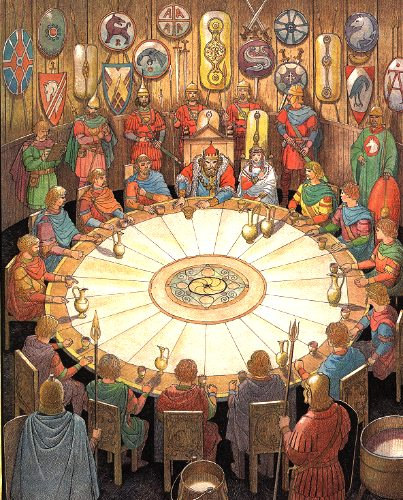# Knights Around A Table

Probability Level 325 of King Arthur's knights are seated at the round table. Three of them are randomly chosen to be sent off to slay a troublesome dragon. Let $P$ be the probability that at least two of the three had been sitting next to each other. If $P$ can be expressed as $\frac {a}{b}$, where $a$ and $b$ are pairwise coprime integers, find $a+b$.

×